# Multilinear form

Jump to: navigation, search-linear form, on a unitary-moduleA multilinear mapping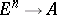(hereis a commutative associative ring with a unit, cf. Associative rings and algebras). A multilinear form is also called a multilinear function (-linear function). Since a multilinear form is a particular case of a multilinear mapping, one can speak of symmetric, skew-symmetric, alternating, symmetrized, and skew-symmetrized multilinear forms. For example, the determinant of a square matrix of orderoveris a skew-symmetrized (and therefore alternating)-linear form on. The-linear forms onform anmodule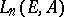, which is naturally isomorphic to the moduleof all linear forms on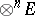. In the case(), one speaks of bilinear forms (cf. Bilinear form) (respectively, trilinear forms).

The-linear forms onare closely related to-times covariant tensors, i.e. elements of the module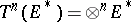. More precisely, there is a linear mapping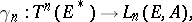such that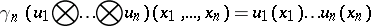for any,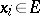. If the moduleis free (cf. Free module),is injective, while ifis also finitely generated,is bijective. In particular, the-linear forms on a finite-dimensional vector space over a field are identified with-times covariant tensors.

For any forms,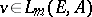one can define the tensor product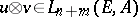via the formula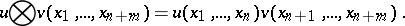For symmetrized multilinear forms (cf. Multilinear mapping), a symmetrical product is also defined:while for skew-symmetrized multilinear forms there is an exterior product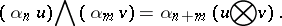These operations are extended to the module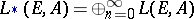, where,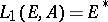, to the module of symmetrized forms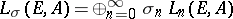and to the module of skew-symmetrized forms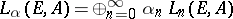respectively, which transforms them into associative algebras with a unit. Ifis a finitely-generated free module, then the mappingsdefine an isomorphism of the tensor algebra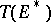onand the exterior algebra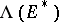on the algebra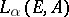, which in that case coincides with the algebra of alternating forms. Ifis a field of characteristic, then there is also an isomorphism of the symmetric algebraon the algebraof symmetric forms.

Any multilinear form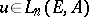corresponds to a function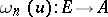, given by the formula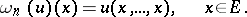Functions of the form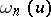are called forms of degreeon; ifis a free module, then in coordinates relative to an arbitrary basis they are given by homogeneous polynomials of degree. In the case() one obtains quadratic (cubic) forms on(cf. Quadratic form; Cubic form). The form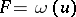completely determines the symmetrization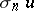of a form: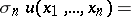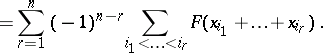In particular, for,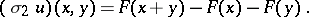The mappingsand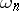define a homomorphism of the algebra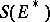on the algebra of all polynomial functions (cf. Polynomial function)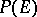, which is an isomorphism ifis a finitely-generated free module over an infinite integral domain.